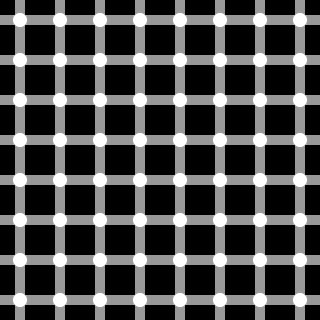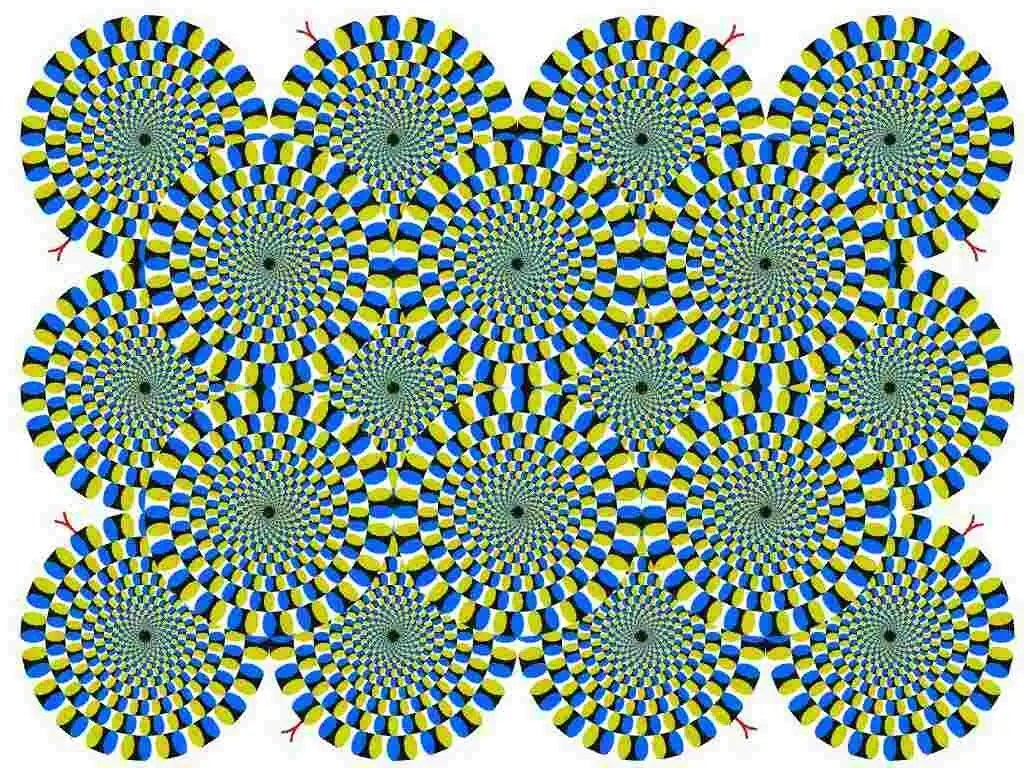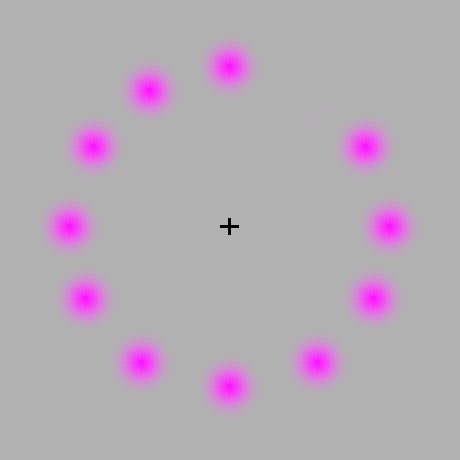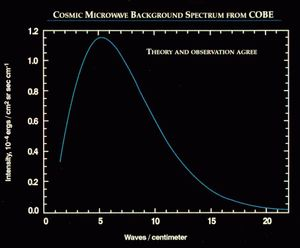# A Candle in the Dark

A look on science, politics, religion and events

## When solitons collide

Adapted from notes I copied during a course I attended

Consider a 1+1 dimensional scalar field$\phi(x,t)$ where x corresponds to the space dimension, and t corresponds to the time dimension. The Lagrangian and the Hamiltonian energy density for the field are given by,$\mathcal{L}=\frac{1}{2} \partial_{\mu}\phi \partial^{\mu}\phi - U(\phi)$$\mathcal{H}=\frac{1}{2}(\partial_0 \phi)^2 + \sum_i\frac{1}{2}(\partial_i \phi)^2 + U(\phi)$

Here, the Einstein summation convention is used, where repeated indices are summed over.$\partial_{\mu}$ is used to denote the four-vector$(\frac{1}{c}\frac{\partial}{\partial t}, \nabla)$

The evolution of this scalar field is governed by the Euler-Lagrange equation,$\frac{\partial \mathcal{L}}{\partial \phi} = \partial_{\mu}\left(\frac{\partial \mathcal{L}}{\partial \left( \partial_{\mu}\phi \right)}\right)$

Plugging in Lagrangian for the scalar field I’ve written above, the E-L equation reduces to,$\Box \phi = -\frac{\partial U(\phi)}{\partial \phi}$

where,$\Box$ is the D’Alembertian. ie,$\Box \phi = \phi_{tt} - \phi_{xx}$

A specific example, where the solutions of the E-L equation have interesting properties, is given by the potential,$U(\phi)=\frac{\lambda}{2} \left(\phi^2-a^2\right)^2$

The lowest energy solutions which satisfy the E-L equations are known as the classical vaccum. In this specific example, they are given by$\phi(x)=a$$\phi(x)=-a$

You can see that these solutions correspond to the minimum of the potential. Additionally, the total energy is also minimized by these solutions, because the contribution of the space and time derivatives to the Kinetic Energy density term is zero.

Apart from these vacuum solutions, there exist finite energy solutions of the E-L equations for this potential. From the criteria that the energy must be finite when the Hamiltonian energy density is integrated over x, such a solution must tend to the classical vacuua solutions at the extremities.

Such a solution is known as the kink solition, and is given by,$\phi(x)=a \tanh{\mu x}$

where,$\mu^2=\lambda a^2$

You can check that this solution satisfies the Euler-Lagrange equation by plugging it in. Note that the solution is time-independent. Furthermore, when$x \rightarrow \pm \infty$,$\phi \rightarrow \pm a$. Therefore, the solution interpolates between the classical vacuua solutions.

To understand why it’s called a kink soliton, let’s plot its functional form, and the corresponding Energy density. For the plots below, I’ve set$a=1, \lambda=1$

As you can see, the solution tends to the vaccum solution (here, a=1) at either end and the energy density appears as a stationary wave packet which is like a kink in a rope.

Now, there are certain global symmetries that Lagrangian has, which are absent in the kink soliton solution. For instance, if we set$x \rightarrow -x$, the Lagrangian in Eq. (1), and consequently the corresponding action, is invariant. However, the solution$\phi(-x) \rightarrow -\phi(x)$, is a new solution and interpolates the other way (ie,$\phi \rightarrow \mp a$ as$x \rightarrow \pm \infty$. This is called the anti-kink solition, and has the same energy as the kink soliton.

There are other symmetries where the Lagrangian is invariant which give rise to new solutions. For example, a spatial translation will give a solution$\phi_{new}=\phi(x-x_0)$, which shifts the location of the center of the kink. A lorentz transformation$x \rightarrow \gamma(x-vt)$ will give a new time dependent solution,$\phi_{new}=\phi\left(\gamma(x-vt)\right)$, where$\gamma=1/\sqrt{1-v^2/c^2}$. This corresponds to a kink which starts out at the origin and moves to the right with a constant velocity.

Therefore, the most general form of the kink soliton can be written as,$\phi_K(x,t) = a \tanh{\mu(x-x_0-vt)}$

This corresponds to a soliton which is initially centered at x_0 and moves with a velocity v to the right. The anti-kink soliton corresponding to this is simply$-\phi_K(x,t)$

Now, consider a box which has both a kink and an anti-kink solition placed away from each other and with velocities in opposite directions. What happens to them as they approach each other? They can’t pass each other, otherwise they’d violate the boundary condition (remember that the kink and anti-kink solitons tend to different vacuum solutions at either end). So you’d expect them to bounce off each other.

In fact, we can even numerically test this, because we know that they are governed by the Euler-Lagrange equation. If$\phi(x,t)$ represents both the kink and anti-kink soliton, then initially, one has$\phi(x,t) = \left\{\begin{array}{c} a\tanh{\mu(x+x_0-vt)} \quad x \leq 0 \\ -a\tanh{\mu(x-x_0+vt)} \quad x \geq 0 \end{array}\right\}$

then, the E-L equation can be rewritten as two first order differential equations$\left(\begin{array}{c} \partial_t \phi(x,t) \\ \partial_t \pi(x,t) \end{array}\right) = \left(\begin{array}{c} \pi(x,t) \\ \partial_x^2\phi(x,t) - \frac{\partial U(\phi)}{\partial \phi}\end{array}\right)$

where,$\pi(x,t) = \partial_t \phi(x,t)$ is the momentum density

To solve the system of equations above, I discretized the spatial derivative using the central difference method, and used a fourth-order Runge Kutta solver with corresponding intial and boundary condition discussed above. You can see how the kink and anti-kink soliton bounce off each other in the video below.

Kinky, ain’t it?

Wikipedia has a gallery of images on solitons for the Sine-Gordon model, where a Sine potential is used instead of the quartic potential. There are also a lot of nice websites on the fantastic properties that such non-linear PDE’s exhibit.

Written by parseval

November 30, 2008 at 6:20 am

Posted in physics, science, videos

## How the leopard may have really got her spots

A mechanism of pattern formation in many animals, (including the zebra, leopard and the giraffe), was first suggested by Alan Turing in 1952.

To understand his mechanism, we’ll first look at a system where two reactive chemical species (morphogens) are present and they do not move about in space(ie, they don’t diffuse). If the concentration of the species are denoted by$u$ and$v$, the rate at which the concentrations change will simply be the rate at which they react. That is,$\frac{\partial u}{\partial t} = f(u,v)$$\frac{\partial v}{\partial t} = g(u,v)$

where,$f(u,v)$ and$g(u,v)$ describe the rate law kinetics which the species obey. If we want to find the steady state concentrations of the species, we simply set the partial derivatives with time as 0, and solve for$u$ and$v$.

What happens if$f(u,v)$ and$g(u,v)$ are non-linear equations with multiple solutions? The stability of each solution can be determined by a linear stability analysis, and the final stable solution will depend on the initial condition. Indeed, because of the homogeneity in the initial constraint, that the initial concentrations of the species are uniform everywhere and the they are spatially fixed, the final concentrations at steady state are again uniform in space.

Linearizing the above system of equations (ie, set$u=u_{ss}+\tilde{u}$ and$v=v_{ss}+\tilde{v})$, one obtains$\left(\begin{array}{c} \frac{d \tilde{u}}{dt} \\ \frac{d \tilde{v}}{dt} \end{array}\right)=\left(\begin{array}{cc} f_u & f_v \\ g_u & g_v \end{array}\right) \left(\begin{array}{c} \tilde{u} \\ \tilde{v}\end{array}\right)$

For the steady states to be stable, the condition is that the real parts of the eigenvalues of the jacobian are negative. For a two component system, this is true when the trace is negative and the determinant positive.

Now, what happens if we remove the spatial constraint and allow the chemical species to diffuse? The equations which describe the concentration of the species will be modified to account for diffusion. We’ll now have$\frac{\partial u}{\partial t} = D_u \nabla^2 u + f(u,v)$$\frac{\partial v}{\partial t} = D_v \nabla^2 v + g(u,v)$

where,$D_u$ and$D_v$ are the diffusivity of the chemical species. One of the observations which Turing made, was that under certain conditions, steady states which were initially spatially uniform could now show spatial variation due to diffusion. The natural question would be, under what conditions would we get these spatial variations? To answer that, we need to delve a bit into linear stability analysis.

However, before doing that, I’ll have to define what the boundary conditions and the geometry of the system is for the second set of equations. A very simple system would be a one-dimensional strip of space, where diffusion and reaction occurs. To make this system ‘self-contained’, we set the flux of the chemical species at the boundary to be 0.

Therefore, the system of equations are$\frac{\partial u}{\partial t} = D_u \frac{\partial^2 u}{\partial x^2} + f(u,v)$$\frac{\partial v}{\partial t} = D_v \frac{\partial^2 v}{\partial x^2} + g(u,v)$

Since we want to find out when the homogeneous solutions are unstable, we need to look at the linearized system in terms of the deviation variables from the steady state solutions,$\tilde{u} = u - u_{ss}$ and$\tilde{v} = v - v_{ss}$. With these variables, the linearized equations are$\frac{\partial \tilde{u}}{\partial t} = D_u \frac{\partial^2 \tilde{u}}{\partial x^2} + f_u \tilde{u} + f_v\tilde{v}$$\frac{\partial \tilde{v}}{\partial t} = D_v \frac{\partial^2 \tilde{v}}{\partial x^2} + g_u \tilde{u} + g_v\tilde{v}$

The solutions to this equation can be shown to be of the form.$\tilde{u} = u^* e^{\sigma t} \sin{\alpha x}$$\tilde{v} = v^* e^{\sigma t} \sin{\alpha x}$

(Why? Substitute and check!)

Substituting to the equation, we get$u^* (\sigma +D_u\alpha^2 - f_u) -f_v v^* = 0$$v^* (\sigma +D_v\alpha^2 - g_v) -g_u u^* = 0$

Here’s the important argument. For a spatially varying pattern, we require non-zero solutions to$u^*$ and$v^*$. The condition for that is$\left|\begin{array}{cc} \sigma +D_u\alpha^2 - f_u & -f_v \\ -g_u & \sigma +D_v\alpha^2 - g_v \end{array}\right| = 0$

This can be re-written as$det( \sigma I - A + \alpha^2 D) = 0$

where,$A= \left(\begin{array}{cc} f_u & f_v \\ g_u & g_v \end{array}\right)$$D = \left(\begin{array}{cc} D_u & 0 \\ 0 & D_v \end{array}\right)$

Now, the value of$\sigma$ is the eigenvalue of the matrix$R= (A - \alpha^2 D)$, and for the homogeneous solution to be unstable, one requires that the real part of the eigenvalues of R are positive for some$\alpha^2$

This condition can be simplified to,$\sigma^2 - Tr(R) \sigma + det(R) =0$

For the eigenvalue to be positive, we require that$det(R)<0$ and$Tr(R)>0$ .

But, since$Tr(R) = Tr(A - \alpha^2 D) = Tr(A) - \alpha^2 Tr(D)$ and$Tr(A)$ is less than 0 because the homogeneous steady state is assumed to be stable,$Tr(R)$ can never be positive. So, the criteria for instability of the homogeneous solution is that$det(R)<0$. One can also relate this to the ratio of the diffusivites of the two species u and v.

This means that under this condition, the homogeneous steady state solution is unstable, and the concentrations of u and v will increase till the non-linear terms we have neglected plays a role and causes spatial patterns.

I will edit and add pretty pictures displaying the simulation of this phenomenon once I figure out how to use the pdetool in MATLAB to solve non-linear parabolic partial differential equations in 2 variables.

References
The Chemical Basis of Morphogenisis, A.M Turing, Philosophical Transactions of the Royal Society of London. Series B, Biological Sciences, Vol. 237, No. 641. (Aug. 14, 1952),pp 37-72

Two-stage Turing model for generating pigment patterns on the leopard and the jaguar, Phys. Rev. E 74, 011914 (2006)

Mathematical Biology, JD Murray, 3rd edn, Springer (2002). chapter 2.4 &2.5

Written by parseval

April 14, 2008 at 4:28 pm

## Free convection for dummies

with one comment

The Nonoscience blog has a nice series of articles that serve as an introduction to the principles of free convection. You can find the posts here:

As for me, I’m still grappling to make sense of my heat transfer course. It’s hard enough trying to get a physical grasp of all those dimensionless quantities1, and on top of that, my head’s still reeling trying to figure out when to use all the empirical correlations2.

Notes

 – Nusselt, Rayleigh, Prandtl, Grashof, Richardson, apart from good old Reynolds and Archimidies. I could probably write a poem!

 – Churchill and Chu, you’re exempt. But Dittus & Boelter, Sieder & Tate, Petukhov, Notter & Sleicher, I’m piping confused. And then there’s Whitaker, Polhausen , … I could go on & on

Written by parseval

April 15, 2007 at 12:24 pm

Posted in physics

## Optical Illusions

These are some really neat optical illusions. Many optical illusions are beautiful, astonishing and powerful. I think they show how easy it is to fool the visual system and the brain.

## Same color illusionIn the above image, look carefully at the squares marked as A and B. One appears white and the other appears black, right?

Amazingly, both the squares have the same color! Don’t believe it?

To verify that the color’s are the same, try cutting the two squares and place them side by side in paint or photoshop. Or, you could use the colorpicker tool to check that the colors are the same.

There’s an explanation of why this illusion works at the MIT site.

## Scintillating Grid IllusionThis is a famous one. You’ll see the sudden appearance of black dots at the crossings. Also, if you keep your eye fixed on a particular intersection, no dots appear there.

I wasn’t able to find any explanation on how this illusion actually works.

## Peripheral drift illusionThe ‘rotating snake’ illusion is one of my favorites. The ‘rotation’ of the wheels occurs relative to your eye movements. It’s thought that asymmetric luminance at the periphery of the vision causes this illusion.

Click on the image to get a larger version of the illusion.

## Afterimage illusionKeep concentrating on the sign at the center of the circle.

Do you see the rotating green spot?

## Fraser’s SpiralActually, there are no spirals!
The intersecting black arcs appear to form a spiral, but they are in fact concentric circles.

## Zollner_illusionParallel or not?

External Resources

Written by parseval

March 8, 2007 at 8:00 pm

Posted in fun, physics

## Physics Nobel

It’s that month of the year when we honour the men and women who represent the pinnacle of human achievement, marvel at their magnificence, applaud their brilliance and … enough with the eloquence. To get to the point, it’s Nobel Prize time!

The Nobel Prize in physics for 2006 was awarded to two Americans, John C. Mather and George F. Smoot “for their discovery of the black body form and anisotropy of the cosmic microwave background radiation.”

What did they do to get the nobel? Their work helped in providing very strong experimental evidence which supports the hot big-bang model of the universe. Mather and Smoot were involved in analysing the data of the COBE satellite, which was placed on orbit on November 18, 1989The picture to the left shows the cosmic microwave background spectrum, carefully measured by the FIRAS instrument on the COBE satellite. In fact, this is the most accurately measured spectrum in nature. The error bars are so small, they can’t be drawn on the graph! Also, the data points can’t be seen, because they fit the theoretical curve so perfectly!

Now, the Cosmic microwave background (CMB) is a consequence of the big bang theory. In the beginning, the universe was a hot plasma full of high energy protons, baryons and what not. It was so dense, that even photons couldn’t travel through. As the universe expanded and coooled, the protons and electrons combined to form neutral atoms, and the photons (ie, light) was able to travel freely. So, the theory says that the radiation emitted at that point, was that of a perfect black body from a spherical surface. Look at the graph of intensity vs wavenumber again. It shows that the experimental data collected by COBE, matches absolutely perfectly with the black body graph.

The ansiotropic effects (property of being directionally dependent) of the CMB were predicted by the theory due to scattering at the surface. This was also succesfully measured by the COBE satellite.

Per Carlson, the chairman of the Nobel committee for physics, said

It is one of the greatest discoveries of the century. I would call it the greatest. It increases our knowledge of our place in the universe.

Written by parseval

October 3, 2006 at 5:40 pm

Posted in nobel prize, physics